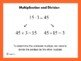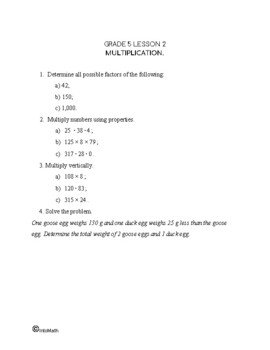CA\$2.00

Division is  the opposite operation to multiplication. When we are trying to distribute something evenly or with a remainder among a group of people or split something into smaller components – we are dividing. We cannot divide by 0, since no splitting or sharing is taking place.

We use the ÷ symbol, or sometimes the / symbol to demonstrate division. The symbol ÷, called  obelus  or obel  (from the Greek οβελοσ), was introduced by the Swiss mathematician Johann Henrich Rhan in 1659.

Knowing the multiplication tables can help with division.CA\$4.00

To divide larger numbers, we need to understand the place value of each digit within each number. Then use the method of long division. In order to set up a long division operation, write the dividend on the right, under the division symbol, and the divisor to the left on the outside. The result of dividing any two numbers by each other is called a quotient and it will go on top of a division symbol. Watch the video above to see a step-by-step demonstration.

Sometimes, numbers do not divide evenly and there is a remainder. The video demonstrates how to divide with a remainder. In order to practice this skill, check out the workbook and complete the exercises.

All possible numbers that a given number is evenly divisible by are called the factors of the given number.

Some numbers are only divisible by themselves and 1, such numbers are called prime factors. Greatest Common Factor (used when working with fractions) is also based on the same concept.

Watch the video lesson, check out the note and practice for additional math help on the topic.